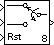Documentation

# N-Sample Switch

Switch between two inputs after specified number of sample periods

## Library

Signal Management / Switches and Counters

`dspswit3`## Description

The N-Sample Switch block outputs the signal connected to the top input port during the first N sample times after the simulation begins or the block is reset, where you specify N in the Switch count parameter. Beginning with output sample N+1, the block outputs the signal connected to the bottom input until the next reset event or the end of the simulation.

You specify the sample period of the output in the Sample time parameter (that is, the output sample period is not inherited from the sample period of either input). The block applies a zero-order hold at the input ports, so the value the block reads from a given port between input sample times is the value of the most recent input to that port.

Both inputs must have the same dimension, except in the following two cases:

• When one input is a scalar, the block expands the scalar input to match the size of the other input.

• When one input is an unoriented vector and the other input is a row or column vector with the same number of elements, the block reshapes the unoriented vector to match the dimension of the other input.

The Reset input check box enables the Rst input port. At any time during the count, a trigger event at the Rst port resets the counter to zero. The reset sample time must be a positive integer multiple of the input sample time. This block supports triggered subsystems when you select the Reset input check box.

You specify the triggering event in the Trigger type pop-up menu, and can be one of the following:

• `Rising edge` — Triggers a reset operation when the `Rst` input does one of the following:

• Rises from a negative value to a positive value or zero

• Rises from zero to a positive value, where the rise is not a continuation of a rise from a negative value to zero (see the following figure)• `Falling edge` — Triggers a reset operation when the `Rst` input does one of the following:

• Falls from a positive value to a negative value or zero

• Falls from zero to a negative value, where the fall is not a continuation of a fall from a positive value to zero (see the following figure)• `Either edge` — Triggers a reset operation when the `Rst` input is a ```Rising edge``` or `Falling edge` (as described above).

• `Non-zero sample` — Triggers a reset operation at each sample time that the `Rst` input is not zero.

## Parameters

Switch count

The number of sample periods, N, for which the output is connected to the top input before switching to the bottom input. Tunable (Simulink).

Reset input

Enables the Rst input port when selected. The rate of the reset signal must be a positive integer multiple of the rate of the data signal input.

Trigger type

The type of event at the Rst port that resets the block's counter. This parameter is enabled when you select Reset input. Tunable (Simulink).

Sample time

The sample period, Ts, for the block's counter. The block switches inputs at t=Ts*(N+1).

## Supported Data Types

• Double-precision floating point

• Single-precision floating point

• Fixed point (signed and unsigned)

• Boolean — The block accepts Boolean inputs to the Rst port, which is enabled when you set the Reset input parameter.

• 8-, 16-, and 32-bit signed integers

• 8-, 16-, and 32-bit unsigned integers

 Counter DSP System Toolbox N-Sample Enable DSP System Toolbox

Watch now